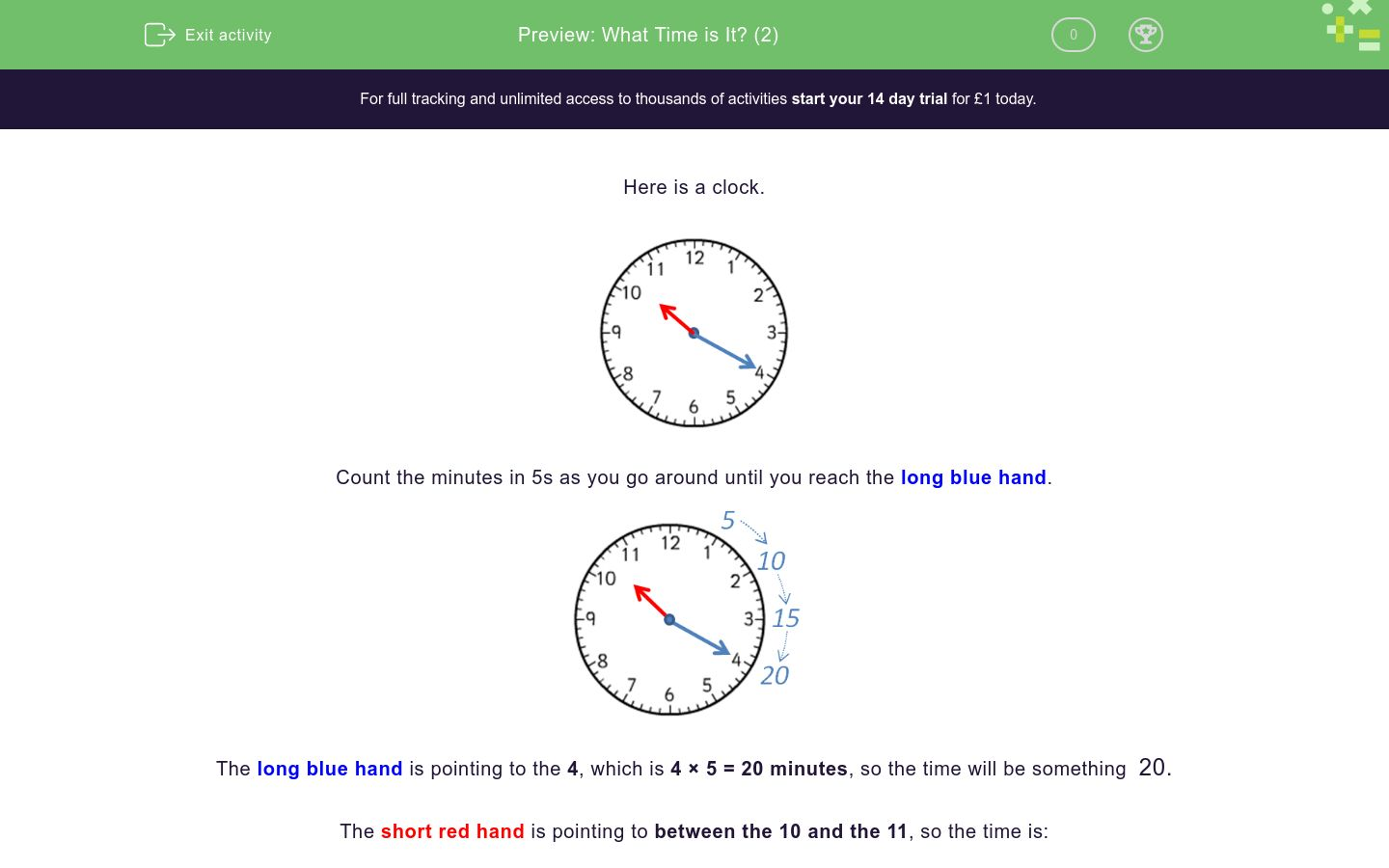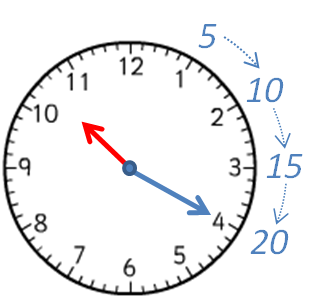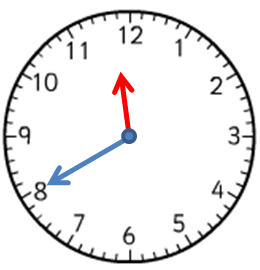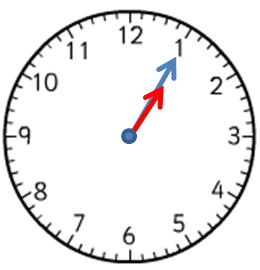# What Time is It? (2)

In this worksheet, students must select the time that matches the given clock face. Times in multiples of 5 minutes are shown, such as 2:35 or 25 to 3.Key stage:  KS 1

Curriculum topic:   Measurement

Curriculum subtopic:   Tell/Write the Time

Difficulty level:### QUESTION 1 of 10

Here is a clock.Count the minutes in 5s as you go around until you reach the long blue hand.The long blue hand is pointing to the 4, which is 4 × 5 = 20 minutes, so the time will be something  20.

The short red hand is pointing to between the 10 and the 11, so the time is:

10:20

or 20 past 10 because it is 20 minutes past 10 o'clock.

Here is another clock.Count the minutes in 5s as you go around until you reach the long blue hand.The long blue hand is pointing to the 7, which is 7 × 5 = 35 minutes, so the time will be something  35.

The short red hand is pointing to between the 2 and the 3, so the time is:

2:35

or 25 to 3 because there are 25 minutes until 3 o'clock.

What time is shown on the clock?10:15

10:05

10:25

What time is shown on the clock?3:10

2:15

What time is shown on the clock?12:40

11:40

11:08

What time is shown on the clock?5 to 8

5 past 8

7:11

7:55

Write the time shown on the clock.

Use a colon : to separate the hour from the minutes (e.g. 7:30).Write the time shown on the clock.

Use a colon : to separate the hour from the minutes (e.g. 7:30).Write the time shown on the clock.

Use a colon : to separate the hour from the minutes (e.g. 7:30).What time is shown on the clock?5:55

4:55

5 to 4

5 to 5

What time is shown on the clock?8 to 8

20 to 7

20 to 8

What time is shown on the clock?2 past 12

10 past 12

10 to 1

• Question 1

What time is shown on the clock?10:25
EDDIE SAYS
The short hand is pointing to between the 10 and the 11.
The long hand is pointing to 5, which is 25 minutes.
• Question 2

What time is shown on the clock?3:10
EDDIE SAYS
The short hand is pointing to between the 3 and the 4.
The long hand is pointing to 2, which is 10 minutes.
• Question 3

What time is shown on the clock?11:40
EDDIE SAYS
The short hand is pointing to between the 11 and the 12.
The long hand is pointing to 8, which is 40 minutes.
• Question 4

What time is shown on the clock?5 to 8
7:55
EDDIE SAYS
The short hand is pointing to between the 7 and the 8.
The long hand is pointing to 11, which is 55 minutes or '5 to'.
• Question 5

Write the time shown on the clock.

Use a colon : to separate the hour from the minutes (e.g. 7:30).09:05
9:05
EDDIE SAYS
The short hand is pointing to between the 9 and the 10.
The long hand is pointing to 1, which is 5 minutes.
• Question 6

Write the time shown on the clock.

Use a colon : to separate the hour from the minutes (e.g. 7:30).01:05
1:05
EDDIE SAYS
The short hand is pointing to between the 1 and the 2.
The long hand is pointing to 1, which is 5 minutes.
• Question 7

Write the time shown on the clock.

Use a colon : to separate the hour from the minutes (e.g. 7:30).01:25
1:25
EDDIE SAYS
The short hand is pointing to between the 1 and the 2.
The long hand is pointing to 5, which is 25 minutes.
• Question 8

What time is shown on the clock?4:55
5 to 5
EDDIE SAYS
The short hand is pointing to between the 4 and the 5.
The long hand is pointing to 11, which is 55 minutes or '5 to'.
• Question 9

What time is shown on the clock?20 to 8
EDDIE SAYS
The short hand is pointing to between the 7 and the 8.
The long hand is pointing to 8, which is '20 to' or 40 minutes past.
• Question 10

What time is shown on the clock?10 past 12
EDDIE SAYS
The short hand is pointing to between the 12 and the 1.
The long hand is pointing to 2, which is 10 minutes past.
---- OR ----

Sign up for a £1 trial so you can track and measure your child's progress on this activity.

### What is EdPlace?

We're your National Curriculum aligned online education content provider helping each child succeed in English, maths and science from year 1 to GCSE. With an EdPlace account you’ll be able to track and measure progress, helping each child achieve their best. We build confidence and attainment by personalising each child’s learning at a level that suits them.

Get started